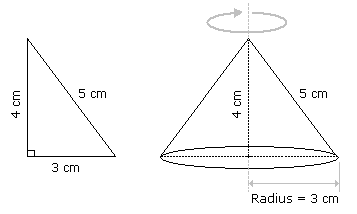# Aptitude - Volume and Surface Area

## Why Aptitude Volume and Surface Area?

In this section you can learn and practice Aptitude Questions based on "Volume and Surface Area" and improve your skills in order to face the interview, competitive examination and various entrance test (CAT, GATE, GRE, MAT, Bank Exam, Railway Exam etc.) with full confidence.

## Where can I get Aptitude Volume and Surface Area questions and answers with explanation?

IndiaBIX provides you lots of fully solved Aptitude (Volume and Surface Area) questions and answers with Explanation. Solved examples with detailed answer description, explanation are given and it would be easy to understand. All students, freshers can download Aptitude Volume and Surface Area quiz questions with answers as PDF files and eBooks.

## Where can I get Aptitude Volume and Surface Area Interview Questions and Answers (objective type, multiple choice)?

Here you can find objective type Aptitude Volume and Surface Area questions and answers for interview and entrance examination. Multiple choice and true or false type questions are also provided.

## How to solve Aptitude Volume and Surface Area problems?

You can easily solve all kind of Aptitude questions based on Volume and Surface Area by practicing the objective type exercises given below, also get shortcut methods to solve Aptitude Volume and Surface Area problems.

### Exercise :: Volume and Surface Area - General Questions

1.

A right triangle with sides 3 cm, 4 cm and 5 cm is rotated the side of 3 cm to form a cone. The volume of the cone so formed is:

 A. 12cm3 B. 15cm3 C. 16cm3 D. 20cm3

Explanation:Clearly, we have r = 3 cm and h = 4 cm.Volume = 1r2h =1 xx 32 x 4cm3 = 12cm3. 3 3

Video Explanation: https://youtu.be/Njsid2mVwUY

2.

In a shower, 5 cm of rain falls. The volume of water that falls on 1.5 hectares of ground is:

 A. 75 cu. m B. 750 cu. m C. 7500 cu. m D. 75000 cu. m

Explanation:

1 hectare = 10,000 m2

So, Area = (1.5 x 10000) m2 = 15000 m2.

 Depth = 5 m = 1 m. 100 20Volume = (Area x Depth) =15000 x 1m3 = 750 m3. 20

Video Explanation: https://youtu.be/dDbVuBzYop8

3.

A hall is 15 m long and 12 m broad. If the sum of the areas of the floor and the ceiling is equal to the sum of the areas of four walls, the volume of the hall is:

 A. 720 B. 900 C. 1200 D. 1800

Explanation:

2(15 + 12) x h = 2(15 x 12)h = 180 m = 20 m. 27 3Volume =15 x 12 x 20m3 = 1200 m3. 3

Video Explanation: https://youtu.be/V8EQ1YIaH74

4.

66 cubic centimetres of silver is drawn into a wire 1 mm in diameter. The length of the wire in metres will be:

 A. 84 B. 90 C. 168 D. 336

Explanation:

Let the length of the wire be h.

 Radius = 1 mm = 1 cm. Then, 2 2022 x 1 x 1 x h = 66. 7 20 20h =66 x 20 x 20 x 7= 8400 cm = 84 m. 22

5.

A hollow iron pipe is 21 cm long and its external diameter is 8 cm. If the thickness of the pipe is 1 cm and iron weighs 8 g/cm3, then the weight of the pipe is:

 A. 3.6 kg B. 3.696 kg C. 36 kg D. 36.9 kg

Explanation:

Volume of iron
 =22 x [(4)2 - (3)2] x 21cm3 7
 =22 x 7 x 1 x 21cm3 7
= 462 cm3.Weight of iron = (462 x 8) gm = 3696 gm = 3.696 kg.